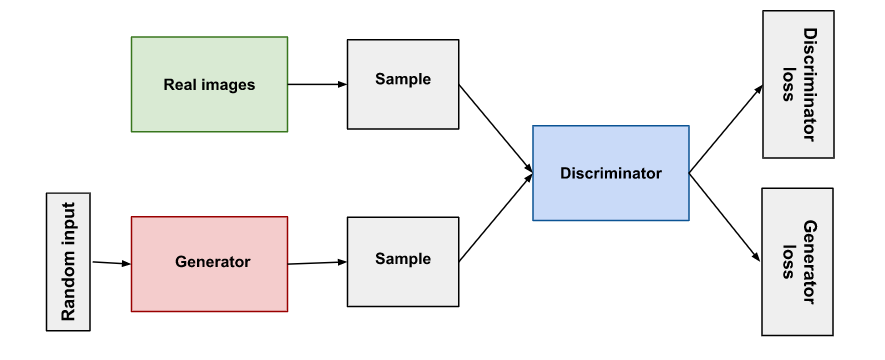# Introduction to Generative Adversarial Networks (GANs)

Original article was published by naim mhedhbi on Deep Learning on Medium# Introduction to Generative Adversarial Networks (GANs)

the model discover and learn the patterns in input data which is used then to generate or output new examples ; generate new examples drawn from the original dataset.

There are two sub-models :

1- the generator model : we train it to generate new outputs / examples .

2- the discriminator model : try to classify examples as real from the domain or fake(generated).

Both the generator and the discriminator are neural networks.

Generative adversarial networks are based on a game theoretic scenario in which the generator network must compete against an adversary. The generator network directly produces samples. Its adversary, the discriminator network, attempts to distinguish between samples drawn from the training data and samples drawn from the generator.

— Page 699, Deep Learning, 2016.

This is an overview of the GAN structure. let’s dive into details oh how it works ✌

1–1 HOW Generator model works :

It takes a fixed-length random vector as input and generates a sample in the domain. the vector is created from a Gaussian distribution.